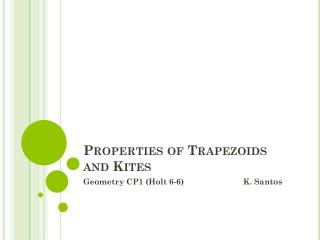DownloadDownload PresentationProperties of Trapezoids and Kites

# Properties of Trapezoids and Kites

Download Presentation## Properties of Trapezoids and Kites

- - - - - - - - - - - - - - - - - - - - - - - - - - - E N D - - - - - - - - - - - - - - - - - - - - - - - - - - -
##### Presentation Transcript

1. Properties of Trapezoids and Kites Geometry CP1 (Holt 6-6) K. Santos

2. Kite Kite—a quadrilateral with exactly two pairs of consecutive sides congruent A B D C

3. Theorem 6-6-1 If a quadrilateral is a kite, then its diagonals are perpendicular. A B D C

4. Theorem 6-6-2 If a quadrilateral is a kite, then exactly one pair of opposite angles are congruent. A B D C <B <D

5. Other Kite Observations --adjacent sides congruent --no parallel sides --1 pair opposite angles congruent --diagonals perpendicular --one diagonal bisects angles formed by adjacent sides --one diagonal is bisected --symmetry about one diagonal --two sets of congruent right triangles

6. Kite Example Find all the missing angles. 50 70

7. Trapezoid Trapezoid—a quadrilateral with exactly one pair of opposite sides parallel A B D C Bases: parallel sides and Legs: non-parallel sides and

8. Isosceles Trapezoid Isosceles Trapezoid—a trapezoid with congruent legs (non-parallel sides) A B D C

9. Theorem 6-6-3 If a quadrilateral is an isosceles trapezoid, then each pair of base angles are congruent. B C A D Given: ABCD is an isosceles trapezoid Then: < B < C < A < D

10. Theorem 6-6-4 If a trapezoid has one pair of congruent base angles then the trapezoid is isosceles. A D B C Given: < B < C Then: ABCD is an isosceles trapezoid

11. Theorem 6-6-5 A trapezoid is isosceles if and only it its diagonals are congruent. B C A D Given: ABCD is an isosceles trapezoid Then:

12. Example—Trapezoid--angles WXYZ is an isosceles trapezoid, m< X = 156. Find m < Y, m < Z and m < W. W Z X Y <X and <Y are congruent base angles So, m < Y = 156 <X and <W are supplementary angles m < W = 180 – 156 So, m < W = 24 <W and <Z are congruent base angles So, m < Z = 24

13. Example—Trapezoid--Diagonals AD = 12x -11 and BC = 9x - 2. Find the value of x so that ABCD is an isosceles trapezoid. A B C D AD = BC 12x – 11 = 9x – 2 3x -11 = -2 3x = 9 x = 3

14. Midsegment of a trapezoid Midsegment of a trapezoid—is the segment whose endpoints are the midpoints of the legs. R S M N U T is the midsegment

15. Trapezoid Midsegment Theorem (6-6-6) The midsegment of a trapezoid is parallel to each base, and its length is one half the sum of the bases. S T M N R U |||| MN = (average of the parallel bases)

16. Example--Midsegment Find x. 8 x 14 x = x = x = 11

17. Example--Midsegment Find EH. F 25 G X 16.5 Y E H XY = 16.5 = 33= 25 + a 8 = a so EH = 8

18. Example—Midsegment (algebraic) Find the length of the midsegment. n + 2 n + 10 3n - 12 n + 10 = 2(n+ 10) = n + 2 +3n – 12 2n + 20 = 4n -10 -2n + 20 = -10 -2n = -30 n = 15 Midsegment: n + 10 = 15 + 10 = 25